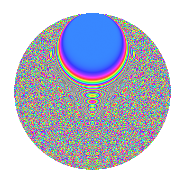# Properties

 Label 320.6.bjLevel 320 Weight 6 Character orbit bj Rep. character $$\chi_{320}(3,\cdot)$$ Character field $$\Q(\zeta_{16})$$ Dimension 1904 Sturm bound 288

# Related objects

## Defining parameters

 Level: $$N$$ $$=$$ $$320 = 2^{6} \cdot 5$$ Weight: $$k$$ $$=$$ $$6$$ Character orbit: $$[\chi]$$ $$=$$ 320.bj (of order $$16$$ and degree $$8$$) Character conductor: $$\operatorname{cond}(\chi)$$ $$=$$ $$320$$ Character field: $$\Q(\zeta_{16})$$ Sturm bound: $$288$$

## Dimensions

The following table gives the dimensions of various subspaces of $$M_{6}(320, [\chi])$$.

Total New Old
Modular forms 1936 1936 0
Cusp forms 1904 1904 0
Eisenstein series 32 32 0

## Trace form

 $$1904q - 8q^{2} - 8q^{3} - 8q^{5} - 16q^{6} - 8q^{7} - 8q^{8} + O(q^{10})$$ $$1904q - 8q^{2} - 8q^{3} - 8q^{5} - 16q^{6} - 8q^{7} - 8q^{8} - 8q^{10} - 16q^{11} + 3160q^{12} - 8q^{13} - 4960q^{14} - 8q^{15} - 16q^{16} - 16q^{17} - 8q^{18} - 8q^{20} - 16q^{21} - 11176q^{22} - 8q^{23} + 20880q^{24} - 8q^{25} - 16q^{26} - 8q^{27} - 8q^{28} - 65144q^{30} - 32q^{31} - 8q^{32} - 512q^{34} - 8q^{35} - 16q^{36} - 8q^{37} - 68832q^{38} - 57904q^{40} - 16q^{41} - 8q^{42} - 8q^{43} + 1936q^{45} - 16q^{46} - 224752q^{48} - 128032q^{50} - 41776q^{51} - 8q^{52} - 8q^{53} - 8q^{55} - 258736q^{56} - 8q^{57} - 195144q^{58} + 97488q^{60} - 16q^{61} - 264q^{62} - 16q^{65} + 254864q^{66} - 8q^{67} + 295264q^{68} - 89280q^{69} - 8q^{70} + 287664q^{71} - 359888q^{72} - 8q^{73} - 8q^{75} - 268496q^{76} - 8q^{77} + 889344q^{78} - 355360q^{79} - 8q^{80} - 16q^{81} + 135832q^{82} - 8q^{83} - 25008q^{85} - 16q^{86} - 564376q^{87} + 622944q^{88} - 8q^{90} - 16q^{91} - 1613848q^{92} - 1952q^{93} + 512q^{94} - 16q^{95} - 16q^{96} + 811232q^{98} + 679040q^{99} + O(q^{100})$$

## Decomposition of $$S_{6}^{\mathrm{new}}(320, [\chi])$$ into newform subspaces

The newforms in this space have not yet been added to the LMFDB.

## Hecke characteristic polynomials

There are no characteristic polynomials of Hecke operators in the database